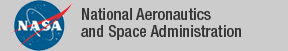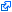### Top File of PVS vectors Library

```
top : THEORY
%----------------------------------------------------------------------------

%
%   Vectors Library V3.0               1/6/2009
%
%   Authors:  Cesar Munoz              National Institute of Aerospace
%             Rick Butler              NASA Langley
%             Ben Di Vito              NASA Langley
%             Hanne Gottliebsen        National Institute of Aerospace
%
%
%   Strategies: vect-distr, vect-distr-off
%
%----------------------------------------------------------------------------
BEGIN
IMPORTING
vectors,         	  % N-dimensional vectors and operations
nvectors,            % N-dimensional vectors based on finite sequences
% See strategies (vect-distr) (vect-distr-off)
vect2D,          	  % Define 2-D Vector from N-dimensional vectors
vect3D,          	  % Define 3-D Vector from N-dimensional vectors

vectors_2D,       	  % 2-dimensional vectors and operations
% See strategies (vect-distr) (vect-distr-off)

vectors_3D,       	  % 3-dimensional vectors and operations
% See strategies (vect-distr) (vect-distr-off)

vectors_4D,       	  % 4-dimensional vectors and operations

vectors_cos,     	  % Law of cosines for n-D vectors
vectors_2D_cos,   	  % Law of cosines for 2D vectors
vectors_3D_cos,   	  % Law of cosines for 3D vectors

distance,        	  % distance function
distance_2D,      	  % 2D-distance function
distance_3D,      	  % 3D-distance function

lines,           	  % Using vectors to define lines, and motion
lines_2D,         	  % Using vectors to define lines, and motion
lines_3D,         	  % Using vectors to define lines, and motion

law_cos_pos_2D,   	  % Law of cosines for 2D positions
law_cos_pos_3D,   	  % Law of cosines for 3D positions

closest_approach,    % calculate t_cpa for moving particles
closest_approach_2D, % calculate t_cpa for moving particles
closest_approach_relative_2D, %
closest_approach_3D, % calculate t_cpa for moving particles

perpendicular_2D,    % line perpendicular to a line through a point
perpendicular_3D,    % line perpendicular to a line through a point
intersections_2D,    % finding intersection points of lines
basis_2D,            % orthonormal basis

matrices,            % Theory of matrices

vect_trig_2D,        % trigonometric properties of 2D vectors
vect_trig_3D,        % trigonometric properties of 3D vectors

cross_3D,            % cross-product
linear_independence_3D,   % linear independence
sigma_2D,            % summations over 2D vectors
sigma_3D,            % summations over 3D vectors
sigma_fseq_3D,
fseqs_ops_vect3,

vect_3D_2D,          % Projection of a 3D vector (x,y,z) into (x,y)
vect_4D_3D_2D,       % Projection of a 4D vector (x,y,z,t) into (x,y,z) and (x,y)
det_2D,              % 2D determinant
parallel_2D,         % 2D parallel
parallel_3D,         % 3D parallel
angles_2D,           % angle of vector
trackAngles_2D,      % track angles (Air Traffic Management)
between_2D,          % defines predicate between?(v1,v2)(u)  EXPERIMENTAL

vect3_basis,
ECEF,                % Earth-Centered Earth Fixed Cartesian coordinate system

vect_fun_ops,        % defines function operators
vect2_fun_ops,
vect3_fun_ops,
linear_transformations_2D, % Linear Functions [Vect2 -> Vect2] AND [Vect2 -> real]

vectors_dot_alt,     % dot product defined using sigma[nat] rather than sigma[below(n)]

test_vec             % test auto_rewrite+ statements

END top

```
The tagidentifies links that are outside the NASA domain